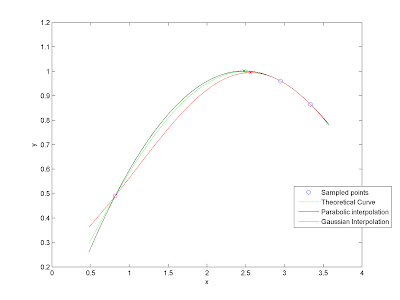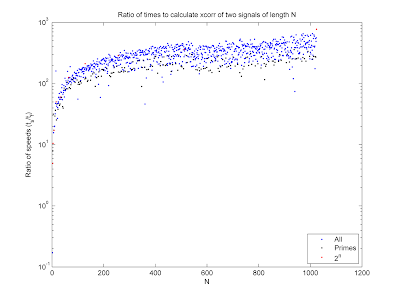## Thursday, June 18, 2009

### Peak EstimationSometimes it is important to be able to estimate the peak of a sampled continuous function between the samples. This is called subsample peak interpolation and is used in radar, delay estimation, and communication. Typically one fits a model to the sampled data and then finds the maximum of the model. Two models that I have used are parabolas and Gaussian curves. Both have three parameters and can be fit exactly to three samples (even if the samples are not evenly spaced), and, as a bonus, closed form solutions exist for parameters. I've written up a Matlab package to demonstrate this procedure, to be found here. The plot above shows an example, finding the peak of the green curve sampled at the blue markers (click to enlarge).

## Friday, June 5, 2009

### Fast Correlation

I've uploaded a small package to the Matlab File Exchange to improve the speed of your cross correlations. This package uses the FFT to calculate the circular cross correlation of two periodic signals. Since the FFT calculates the DFT of a N-point signal in O(N log N) calculations (rather than O(N2)) it is much faster than the naïve method, especially for long signals. In Matlab, the fft method is faster than the multiplication method in all but the most trivial cases, as shown in the image, plotting the ratio of the speed of the fast method to that of the naïve method from the definition. The figure also shows that the fft algorithm is typically fastest when N is a power of 2, and slowest when N is prime.